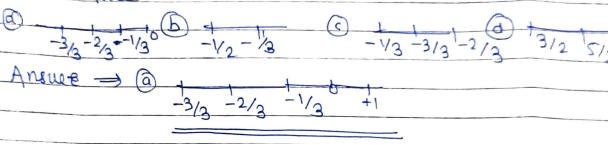# Rational Numbers MCQ Questions Class 8 Maths Chapter 1

Rational Numbers MCQ Questions Class 8 Maths Chapter 1

NCERT MCQ Questions for Class 8 Maths Chapter 1 Rational Numbers. Rational Numbers MCQ Questions with Answers from Class 8 Mathematics.

## Rational Numbers MCQ Questions Class 8 Maths Chapter 1

Rational Numbers Multiple Choice Questions with Answers Class 8 Maths Chapter 1 PDF is available.

Question 1:

Using appropriate properties find?

-4/3 × 3/2 + 8/3 × 9/2 + 16 =?

(a) -26

(b) 26

(c) 23

(d) -24

Ans:

-4/3 × 3/2 = -2, 8/3 × 9/2 = 12

-4/3 × 3/2 + 8/3 × 9/2 + 16

= -2 + 12 + 16

= 26 (b)

Question 2:

(a) 7/13

(b) 13

(c) -13

(d) 0

Ans:

(a), 7/13 is the additive inverse -7/13 because

-7/13 + 7/13

= -7+7/13

= 0/13

= 0 (a) 7/13.

Question 3:

Find the multiplicative inverse of -17

(a) +17

(b) 289

(c) -289

(d) -17

Ans:

17 is the multiplicative  inverse of -17

17 x (-17)

= -289 (a) +17

Question 4:

Solve (-13/27) × 12/17 = 13/17 × -12/27

(a) -156/459

(b) 156/459

(c) 459/156

(d) -156/289

Ans:

(-13) × 12/27×17

= 13 × (-12)/17 × 27

= -156/459

Question 5:

Multiply 7/13 by the reciprocal of -7/16

(a) -16/13

(b) +16/13

(c) 3/16

(d) -13/16

Ans:

Reciprocal of -7/16 is 16/-7

16/-7 × 7/13

= -16/13 (a)

Question 6:

Fill in the blanks:

______ has no reciprocal.

(a) One

(b) Zero

(c) Two

(d) Three

Ans:

(b) Zero.

Question 7:

13/6 is the reciprocal of ——

(a) 6/13

(b -13/6

(c) -6/13

(d) 0

Ans:

(a) 6/13 is the reciprocal of 13/6

Question 8:

Find 2/7 × 7/18 + 4/9 × 9/36 =?

(a) 3/2

(b) 1/3

(c) 2/9

(d) 9/2

Ans:

2/7 × 7/18 + 4/9 × 9/13

= 1/9 + 1/9

= 2/9 (c)

Question 9:

Find 2/13 × 13/16 × 4/5 × 15/16 × ½ =?

(a) 4/89

(b) 2/83

(c) 64/3

(d) 3/64

Ans:

2/13 × 13/16 × 4/5 × 15/16 × 1/2

= 1/8 × 3/4 × ½

= 3/64 (d)

Question 10:

Find 2/5 × (-3/8) + 3/5 × 15/16 – 1/4 =?

(a) 9/13

(b) -13/80

(c) 13/80

(d) 80/13

Ans:

2/5 × -3/8 = -3/20, 3/5 × 15/16 = 9/16

2/5 × -3/8 + 3/5 × 15/16 – -1/4 = -3/20 + 9/16 – 1/4

(c) 13/80 = -192 + 720 – 320/1280

= 208/1280

= 13/80

Question 11:

Find the rational number between 2/3 and 1/2.

(a) -7/12

(b) 7/12

(c) 5/3

(d) 6/3

Ans:

(b) 7/12

(2/3 + ½) ÷ 2

= (4+3/6) ÷2

= 7/6 × ½

= 7/12

7/12 lies between 2/3 and ½

Question 12

Choose rational number greater than -3

(a) -3

(b) -4

(c) -5

(d) -1/2

Ans

(d) -1/2 is the rational number greater than -3.

Question 13:

Represent the -1/3, -2/3, -3/3 on the number lineQuestion 14:

Write the additive inverse of -6/13

(a) 6/13

(b) 13/6

(c) -13/6

(d) 6/5

Ans:

(a) 6/13 is the additive inverse of -6/13

6/13 + -6/13

= 6-6/13

= 0/13

= 0

Question 15:

Solve -8/9 × 9/16 + 4/30 × 15/16 =?

(a) -3/5

(b) 5/3

(c) 8/3

(d) -3/8

Ans:

(d) -3/8, -8/9 × 9/16 = -1/2

4/30 × 15/16 = 1/8

-8/9 × 9/16 + 4/30 × 15/16

= -1/2 + 1/8

= -4+1/8

= -3/8

Updated: April 11, 2023 — 12:13 pm